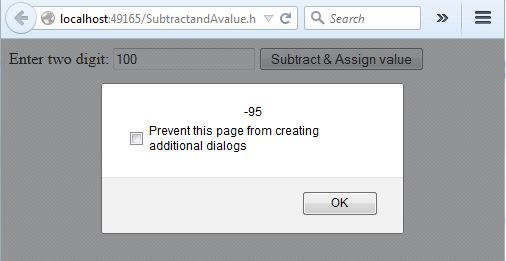#### JavaScript > Assignment Operators

##### How to subtract and assign value in JavaScript?

To subtract and assign value in JavaScript, we can follow this approach.

```  <script>
function Subtractassignvalue() {
var a = document.getElementById("txtA").value;
var result = 5;
result -= parseInt(a);
}
</script>

Enter two digit:
<input type="text" id="txtA" name="txtA" />
<input type="button" name="btnSubtractassignvalue" value="Subtract & Assign value"  onclick="Subtractassignvalue()" />
```

In the above code snippet, we have one text box. On click of button, we are calling the `Subtractassignvalue`function that subtracts and assign the number and show result in the alert. Here also we are parsing the value of text box as Integer and subtracting the way we had done in Add and assign.

OUTPUTViews: 4963 | Post Order: 20